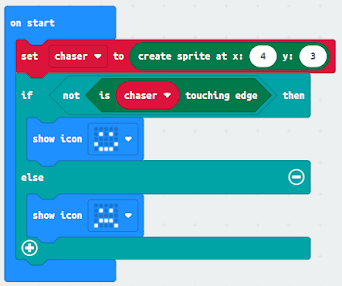Quiz - Microbit Chase the Dot
Which of these blocks will detect when you shake the Microbit? *
10 points
Which of these is the code that let's you use a Microbit like a normal dice? *
10 points
If you rolled two dices at the same time and you added the numbers together, what is the smallest number you could roll? *
10 points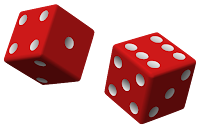If you rolled two dices at the same time and you added the numbers together, what is the largest number you could roll? *
10 points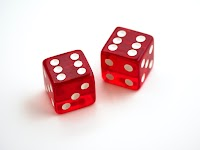Which of these is the code that let's you use a Microbit like throwing 2 normal dice together? *
10 points
In this code we are creating a sprite. What are we storing the sprite in? *
10 points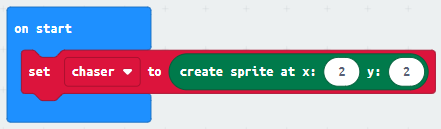This image shows all the possible x and y coordinates of a sprite on a Microbit. Which of the code blocks would you use to set the sprite in the center of the Microbit? *
10 points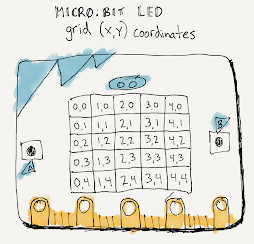If you want to move the 'chaser' sprite to the left, which of these code blocks would you use? *
10 points
What icon will this code show? *
Note the x and y coordinates of the sprite.
10 points